AP State Syllabus AP Board 7th Class Maths Solutions Chapter 8 Congruency of Triangles Ex 3 Textbook Questions and Answers.

## AP State Syllabus 7th Class Maths Solutions 8th Lesson Congruency of Triangles Exercise 3Question 1.
In following pairs of triangles, fmd the pairs which are congruent? Also, write the criterion of congruence.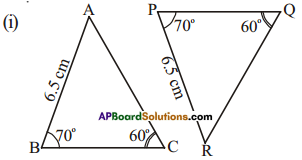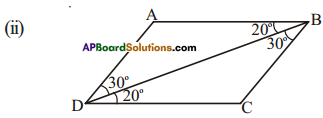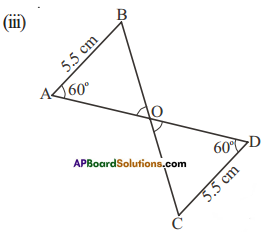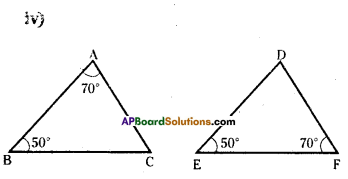Solution:AB = PR
∠B = ∠P
∠C = ∠Q
Now by angle sum property
∠A = ∠R
∴ By A.S.A, ΔABC ≅ ΔRPQ∠ABD = ∠CDB
DB = DBAB = CD
∠A = ∠D
∠B = ∠C (by angle sum properly
By A.S.A
ΔABO ≅ ΔDCOWe can say whether the triangles are congruent or not, at least one pair of sides should be given equal.Question 2.
(i) Are ΔABC and ΔDCB congruent?
(ii) Are ΔAOB congruent to ΔDOC’?
Also identify the relation between corresponding elements and give reason for your answer.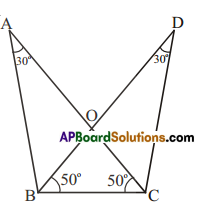Solution:
i) yes.
∠ACB = ∠DBC
BC = BC
∠ABC = ∠DCB (by angle sum property)
∴ ΔABC ≅ ΔDCB (A.S.A)ii) yes
∠A = ∠D
∠ABO = ∠DCO (angle sum property)
AB = DC [From (i)]
∴ ΔAOB ≅ ΔDOC (A.S.A)
Otherwise ΔAOB and ΔDOC are similar by A.A.A. In congruent triangles corresponding parts
are equal.